# Chapter 7 Greek Twilight Galileo

## Transcription

Chapter 7 Greek Twilight Galileo
```88
Chapter 7
Greek Twilight
In questions of science the authority of a thousand is not worth
the humble reasoning of a single individual—Galileo
As we ended the last chapter, the Romans were about to conquer Cartage, and within
150 years of Archimedes' death, the whole Mediterranean world had fallen to Roman
armies. While very able in engineering and law, the Romans did not contribute as
meaningfully to mathematics, and Alexandria would remain the major center of
mathematical activity throughout the Roman Republic and Empire. However, the
concentration of genius that occurred during the first century of Alexandria will not occur
again. Hence, the mathematicians in this extended period—which covers roughly 500
years—will come interspersed through time. Yet even Greek twilight is far brighter than
the mathematical darkness that will encompass Western Europe for the next 700 years.
Alexandrian mathematics ends with the death of Hypatia in the year 415 when she is
murdered by a mob that would not tolerate her pagan beliefs. We will consider 4
mathematicians of this late Alexandrine period: Heron, Ptolemy, Diophantus and
Pappus.
Heron
Heron was a major scientist and mathematician with an extensive bibliography. He is
said to have founded the first College of Technology in Alexandria. One of his major
works, Metrica, was not found until 1896. Not much is known about Heron—not even
exactly when he lived except that he definitely followed Archimedes and definitely
preceded Pappus. We will place him about 105 BC, but scholars have also placed him to
have lived some time during the first two centuries of our era.
Some authors have judged Heron harshly as not having the mental rigor that was
exemplified by Eudoxus and Archimedes. They would blame him for not knowing the
difference between a good approximation and the answer. Yet there is a subtle
philosophical perspective that is stressed in this criticism—namely a Platonic perspective
that would definitely commit to the existence of, for example 2 , while in a different
perspective all one can ever do is approximate it, never realize it, hence a good
approximation is all one will ever achieve, and hence is as good as the answer.
We are going to look at two contributions of Heron. The first one is traditionally called
Heron's formula, but it has been argued that it is due to Archimedes. In any case it is a
wonderful formula, and certainly Heron, if not the originator, is a propagator of it.
89
We all know that the area of a triangle is one half of the base times
the height. But if surveying was our business, calculating the
height may well take time and care. It would be more
advantageous to have a formula that produces the area from
c
b
the lengths of the sides. Heron's formula is such a formula. The
derivation below is not Heron’s; it rather uses elementary but
powerful algebraic techniques that were not available to him. Take
C any triangle with vertices A , B and C and sides a , b and c
B
a
respectively. By dropping the perpendicular
A
from A to BC we get the lengths x , y and z which satisfy:
c
x+ y = a ,
2
2
2
c
d
b
x +z =c
z
2
2
2
e
and
y +z =b .
az
y
Then the area A of the triangle is given by A =
. We proceed
x
C
B
2
a
to eliminate unknowns from the equations in attempting to have just
the lengths of the sides in the expression for the area—namely, we just want a , b and c
in an expression for A .
A
From e, we have that y = b 2 − z 2 (note that the negative sign in front of the square
root would address the oblique triangle case). Substituting in the other two equations, we
have that x + b 2 − z 2 = a and x 2 + z 2 = c 2 , and the y has been eliminated.
From c, we obtain, x = a − b 2 − z 2 , and substituting in x 2 + z 2 = c 2 , we have
a 2 − 2a b 2 − z 2 + b 2 − z 2 + z 2 = c 2 ,
and so 2a b − z = a + b − c , and so b − z =
2
2
2
2
2
2
2
(a
2
+ b2 − c2 )
2
4a 2
4a 2 b 2 − ( a 2 + b 2 − c 2 )
. Hence,
2
z2 =
Since A 2 =
a2 z 2
4a 2
.
, we obtain 16 A 2 = 4a 2b 2 − (a 2 + b 2 − c 2 ) . It is time to pursue a
2
4
symmetric expression on the 3 letters, and since the right hand factors easily, we have
16 A 2 = 2ab + (a 2 + b 2 − c 2 ) 2ab − (a 2 + b 2 − c 2 ) ,
(
)(
)
which can easily be rearranged into
16 A 2 =
((a + b) − c ) (−(a − b) + c ) = (a + b + c)(a + b − c) (−a + b + c)(a − b + c)
2
2
2
2
which is symmetric in the three letters. If we let s denote the semiperimeter of the
a +b+c
triangle, s =
, then we get that
2
a + b + c = 2s , a + b + c = 2s , a + b + c = 2s , a − b + c = 2s − 2b ,
90
a + b − c = 2s − 2c and −a + b + c = 2s − 2a .
16 A 2 = 2 s (2( s − b))(2( s − c))(2( s − a))
A 2 = s ( s − b)( s − c)( s − a )
Thus
and we get
A = s( s − b)( s − c)( s − a).
or equivalently,
Either of the last two expressions is called Heron's formula.
As observed before, note that the symmetry of the three sides of the triangle is reflected
algebraically in the formula since regardless how one permutes a , b and c , s remains
unchanged, and so does A as it should be from the geometry.
The oblique triangle case is just as easily handled.
A
The other contribution of Heron that we consider
has to do with the following geometric problem.
Given two points A and B not on a line, what is the shortest path
from A to B that touches the line?
A
In other words, of all possible paths from A to the
line and from the line to B , which is the shortest?
The answer turns out to be both beautiful and
simple. First find the point C that is the reflection
of the point B on the line. Equivalently, the line
B BC is perpendicular to the given line, and the
distance from B to the line (in other words, its
distance to O ) is the same as the distance from C
to
the line.
A
Then the shortest path is the one through P where
P is the point of intersection of the line AC with
the original line.
P
B
O
C
A
R
P
B
A
B
O
C
A
The reason for this path being the shortest one is
Q
simple. Consider any other path and let Q be the
point where the path intersects the line. Then the
distance from Q to B is the same as the distance
from Q to C since the triangles ΔQOC and
C
ΔQOB are congruent right triangles ( OC ≈ OB
and OQ ≈ OQ ), but then the distance from A to Q and from Q to
B is longer than the path through P since A to P and P to C is a
straight line.
B
O
Note that the path through P is exactly the one that makes the
angle of reflection equal to the angle of incidence: ∠APR = ∠BPO
B
O
91
since the triangle ΔQBC is isosceles and QO is the perpendicular bisector to the base.
This important equality of angles is often referred to as the Law of Reflection, and light
obeys it as it reflects off a flat mirror.
The Greeks were definitely interested in Optics and several authors wrote treatises on
the subject—including Euclid and Ptolemy. Archimedes, in the fanciest of legends, is
reputed to have used huge mirrors to burn Roman ships during the siege of Syracuse.
While the technology to build such a mirror may not have been available, the
mathematical knowledge was certainly within his grasp.
It is even perhaps possible that the Greeks got interested in tangent lines to the conics
because of an interest in mirrors and light reflecting off them. As we mentioned above:
light bounces off a flat mirror by taking the shortest possible path
from one point to the other,
but what happens if the mirror is not flat? How does the ray of light bounce off? This is
where the tangent line comes in. The ray bounces off the tangent line by the law of
reflection—in other words, the angle of incidence with the tangent line to the curve at the
point of contact with the mirror is the same as the angle of reflection with the tangent. Or
equivalently, it goes from one point to the other by the
shortest possible path from one to the other through the
tangent line.
For an example, we will work out what happens in an
elliptical mirror. In our argument we will use the following
intuitive fact: we know the ellipse contains all points whose
distance to the foci is a given constant. Suppose we take a
point outside the ellipse? What is the total distance from that
point to the two foci? Clearly, since we are walking two legs of
P
a triangle, it is greater than from the ellipse itself.
Consider now an ellipse with foci A and B , and consider a
point P on the ellipse. How do we find the tangent line to
A
B
the ellipse at the point P?
Among all possible lines through P , which one is the
tangent?
P
A
B
By Heron's result and the intuitive fact mentioned above,
the answer is easy: since the
Y
shortest path from A to B using
P
the tangent line is the path X
through P , we must have
∠APX = ∠BPY ,
A
B
which means that when a beam of light is lit at one focus in
an elliptical mirror, it shines at the other focus.
92
Ptolemy
Ptolemy (c.170 AD) has an indelible name in the history of astronomy. His masterpiece
in the subject, which we know mainly by its Arab name of the Almagest (or Great
Work), was the reference book on the subject for over 12 centuries. We hear much, and
deservedly so, about the Copernican revolution in astronomy, however, Ptolemy's
humility in his claims should be pointed out.
• First, although Aristarchus (from Athens) had proposed a heliocentric system,
Ptolemy adopted a geocentric one because this approach was prevalent in his time
(mainly due to Aristotelian influence), and he observed that this prevalent influence
was one of the reasons for the geocentric view.
• Second, he was committed to circles partly because of Platonic influence, and hence
he used epicycles, or epicycles of epicycles, etcetera. (up to 27 different types)⎯
which will be clarified briefly. Actually, Copernicus himself believed in the circular
path of the planets around the sun. This is not a great aberration since although we
know today the paths to be elliptical; these are ellipses that resemble circles.
• Finally, Ptolemy emphasized that all he had in his book was a system that worked—
and indeed it did for over a thousand years, and no implication of truth was ever
made. He indeed was much wiser (and humbler) than many of his followers who
insisted on his views as being without the possibility of error.
We will start our discussion of
Ptolemy by describing briefly
what an epicycle is—we will
not use them afterwards at all.
In order to justify the
movement of the astral
bodies, but using only circles,
epicycles were created. The
Epicycles
basic epicycle functions as
follows: consider the path of a
point going around a circle with uniform velocity, where simultaneously the center of the
circle is going around another circle with uniform velocity. There were many types of
basic epicycles depending on the radii and relative velocities of the centers. We have
given examples of two. If that was not enough we could have a point going around a
circle whose center is going around a circle whose center is going around a circle,
etcetera.
Mathematically, Ptolemy's greatest contribution was a first-rate trigonometric table.
More accurately, he left behind a table of chords. As any other astronomer, Ptolemy
93
was interested in solving triangles, which means that if we are given 2 sides and an angle,
or two angles and a side, or 3 sides, of a triangle we must be able to solve for the other
angles and sides. And as is today, the trigonometric functions are invaluable for this
purpose. Actually, the Greeks did not consider our present day functions; rather they had
only one function called the chord of an angle. Namely, given an angle α , chord α is
the length of the chord subtended by α in a given circle.
chordof α
Ptolemy used circles of radius 60 (reminiscences of Babylon) and
used the hexagesimal notation for writing his decimal numbers,
thus 3;15,30 indicated 3+
15
60
+
30
3600
α
60
where we use the semicolon
to indicate the decimal point.
For example, then, chord 60°=60 since the chord is the radius. On the other hand, by
using the Pythagorean theorem, chord 90°= 60 2 . Ptolemy would use the old
Babylonian approximation of 1; 24,51,10 for 2 which is correct to 6 decimal places.
A
chordof 72
C
Let us compute chord 72°, which is precisely the length of the
side of the pentagon. From the Athenian construction, we know
6
0
O 30 B
BC = AB = 602 + 302 , and thus CO = 30 5 − 30 , and finally,
we get AC = chord 72 = 30 10 − 2 5 , which Ptolemy wrote
in the form 70;32,3.
chordof 180 −α
Thus Ptolemy set out to compute a table of chords. What tools
did he use? One of the tools was easily gotten from Thales'
Theorem,
α
60
chord(180°−α ) = 120 2 − (chord α ) 2 ,
But there were other tools he used extensively, and some were
based on what is often called Ptolemy's Theorem. The main tool in proving this theorem
is Proposition 20 of Book III of Euclid, which was proven in the previous chapter:
A
any two angles subtended by the same chord are equal.
D
Now, we have
Theorem. Ptolemy. Let the quadrilateral ABCD be
cyclic, in other words, inscribed in a circle. Then
(AB)(CD)+(AD)(BC)=(AC)(BD) .
Proof. Find the point E so that ∠EAD = ∠BAC . Then the triangles
ΔBAC and ΔEAD are similar since ∠EAD = ∠BAC and
∠BDA = ∠ACB since they subtend the same chord AB . Thus,
E
B
C
94
ED AD
, or equivalently, ED × AC = AD ×BC . Concurrently, ΔBAE is similar to
=
BC AC
BE AB
ΔCAD since ∠CAD = ∠BAE and ∠ABE = ∠ACD , and thus
, or
=
CD AC
BE × AC = AB × CD .
Since BE + ED = BD , by adding the two equations we obtain the theorem.
a
The theorem was the crucial step to one of the two weapons that Ptolemy used the most.
If one knows the chord of two angles, does one know the chord of
the sum of the two angles?
And
if one knows the chord of an angle, does one know the chord of half
that angle?
To both of these questions we can answer YES, and both solutions can be gotten from
Ptolemy's theorem (which of course is much older than Ptolemy).
First we can use Ptolemy's theorem
to compute the chord (90°−α )
given that we know the chord α .
So in the picture we know AB , and,
of course we know AD ( = 120 ).
Also, by Thales' Theorem, BD is
known and so are CD = AC = 60 2 .
Hence by Ptolemy's theorem, since
AD×BC + AB ×CD = BD× AC , we can know BC , the chord (90°−α ) .
Suppose α and β are given, and
suppose their chords are given.
How do we find chord(α + β) ?
By the previous remark, we know
the chords of 90°−α and
and
since
the
90°− β ,
complementary angle is α + β , we can see that in the picture, we seek to compute the
length BC ( = chord(α + β) )
while we know AB , CD , AD .
By Thales' theorem we also know
AC and BD , and thus the only
A unknown quantity in Ptolemy's
D
Theorem is BC .
95
To obtain the chord of half an angle, we reason as follows. We know AB ( chord α ) and
OC = OB = OA = 60 . We also know that where the chord AB intersects the radius OC is
the midpoint of the chord AB , and we also know they
intersect at a 90° angle. Let D be this point of intersection.
Since we know AD and OA , we know OD , and since we
know OC and OD , we know DC , but then we can know
AC , and that is the chord we desire.
Ptolemy used these two steps over and over to build his
table. For example, since he had chords for 60° and 72°, he
could find 12°, and then 6°, and 3°, etcetera.
c
δ
But what does one really need in order to solve a triangle? For example,
a suppose that in the given right triangle we are given the hypotenuse c and
the angle δ . What do we do in order to find the side a ?
Putting the triangle in a circle, it becomes clear that, by similarity of triangles,
c
is the
60
2a
. Or equivalently,
chord (2δ )
chord(2δ ) c
a=
× ,
2
60
and thus half the chord
of twice the angle
became an important
function—in fact, more
important than the chord,
and thus the Hindus (and
the Arabs) built tables not
of chords, but of this new
expression, which we know as the sine function, a name it derived in the West through
mistranslation.
same as
Diophantus
Many consider Diophantus (c.250 AD) one of the original algebraists, although it is the
Arabs that give us both the word algebra and a more refined art for it. In fact,
Diophantus uses a symbol for the unknown, and symbols for its powers, which he
considers all the way to the sixth power—the consideration of any power above three
represented a more sophisticated view than those in the Greek beginnings, but remember,
by now, more than 500 years have passed since Euclid.
96
He also introduces equations, and their elementary manipulations. His notation is all very
different from ours, yet the ingredients are there. Most of our modern algebraic notation
comes from much later, the fifteenth and sixteenth centuries, and its mainly Western
European in origin.
Diophantus' masterpiece is called the Arithmetica, a book not on arithmetic, but on what
we would today call number theory, although primes and divisibility are not discussed
anywhere. The Arithmetica will be translated into French in the seventeenth century, and
through it influence mathematics considerably. In particular, Fermat, the best French
mathematician of that century is going to read it and comment on it considerably.
The Arithmetica consists of a list of problems that are to be solved with rational numbers.
As a matter of fact, the words diophantine equations have come to mean equations that
are to be solved in integers, but it is easy to go from equations to be solved in rationals to
equations that are to be solved in integers. However, the techniques may differ
considerably.
We will look at two problems in the Arithmetica. They both emphasize the use of algebra
as an art where one thinks carefully about technique. This attitude is rarely stressed
nowadays, yet it should. Since he worked with only one unknown, he had to be careful
about what he chose to be that unknown. Both of the next two examples illustrate this
cleverness, where we would normally jump at two unknowns, he made do with one.
One problem would be easily handled today, but perhaps not as elegantly as Diophantus:
Suppose two numbers are given where their sum is 20 and their
product is 96. Find the numbers.
Let x be the amount by which the larger number exceeds 10. Thus, the larger number is
10 + x , and so the smaller number is 10 − x . But then their product is 100 − x 2 which is
supposed to be 96, and hence, x = 2 , which gives the solution 12 and 8.
He would not allow irrational numbers, and, so, for example, if the product had been 97
instead, he would have announced no solution to the problem, since x 2 = 3 does not have
rational solutions.
A modern problem in the same vein:
If Rita gives Tony \$30, then Tony would have twice as much money
as Rita would. On the other hand, if Tony gives Rita \$50, then Rita
would have 3 times as much money as Tony would. How much
money does each have at present?
Let x be the amount of money Rita has left if she gives Tony the \$30. But then, Tony
would have 2x . On the other hand, if Tony gives the \$50 instead, then he would have
2 x − 30 − 50 = 2 x − 80 while Rita would have x + 30 + 50 = x + 80 , and we know that
the amount Rita has is three times the amount that Tony has. Thus, x + 80 = 3(2 x − 80) ,
97
which translates into 5 x = 320 , or x = 64 , and so Rita started with \$94, while Tony
started with 2 x − 30 = \$98 .
We look at another problem in the Arithmetica:
To break 13 into two squares, both of which are greater than 6.
Notice this is an indeterminate problem in that it does not have a unique solution, and
Diophantus is not particularly interested in finding all solutions, just one. However, that
solution has to consist of rational numbers. In modern notation, we want a and b such
that
a 2 + b 2 = 13, a 2 > 6, b 2 > 6 .
In modern terms, Diophantus is interested in finding a rational point in the circle
x 2 + y 2 = 13 that is near the y = x -line. Although we will adopt a modern approach to
his quest, many authors feel that except for the difference in notation (and our level of
comfort with irrationals), the ideas were Diophantus’.
The point (3,2) is a rational point in the circle. Any line with rational slope going
through this point will intersect the circle at another rational point. Since we want to end
near the 45° -line, we should aim at the point ( 6.5, 6.5 ) , so the slope should be a
rational number close to
6.5 − 2
6.5 − 3
≈ −1.219 . Thus we can try slope − 65 . The line going
through (3,2) with slope − 65 is the line 6 x + 5 y = 28 , and searching for the intersection
of this line with the circle, we obtain, by substitution for y =
28 − 6 x
5
, the following
quadratic, 25 x 2 + 784 − 336 x + 36 x 2 = 325 , which simplifies to 61x 2 − 336 x + 459 = 0 ,
and readily solves into x =
possible x), and so y =
158
61
168 ± 15
61
, and so we have x =
153
61
(as the other
, and the problem has been solved since x 2 > 6 .
But, perhaps the oldest objects of interest in number theory are Pythagorean
triples, of which the ancient Mesopotamians left behind a table as we saw
before. Indeed the search for triples of positive integers a , b and c such that
a 2 + b 2 = c 2 , for whatever reason, is old.
When we discussed the Mesopotamians, we mentioned a procedure for producing triples.
Let α and β be relatively prime positive integers, with α > β . Also one may assume
(exactly) one of them is even. Let
a = α 2 − β 2 , b = 2αβ and c = α 2 + β 2 ,
then a , b and c is a Pythagorean triple. Moreover, any triple can be obtained this way.
Following Diophantus, we adopt a slightly different view. Rather than looking for
positive integer solutions to the equation a 2 + b 2 = c 2 , one could divide by c 2 and
98
switch the point of view and consider rational solutions to the equation x 2 + y 2 = 1 . One
can then see that such solutions correspond to Pythagorean triples and vice versa, any
Pythagorean triple a , b and c corresponds to the rational solution x =
a
c
b
and y = . For
example, the triple 3, 4 and 5 would correspond to the rational solution x =
c
3
5
and y =
4
.
5
This is similar to the approach in the previous problem from the Arithmetica.
Geometrically what are we doing? We are looking for rational points on
the unit circle—more accurately, for nonnegative rational points on the
unit circle. Consider the point (− 1,0) , which is on the circle. If we draw
any line with rational slope going through this point, the line will
(− 1,0 )
intersect the circle at another rational point. This is because once a
quadratic equation with rational coefficients has one rational root, then
it must have another—since the sum of the roots is the coefficient of x ,
or because the product of the roots is the constant term. Conversely, the line going
through any rational point in the circle and the point (− 1,0) will have rational
coefficients.
Thus we consider an equation of the form y = mx + m (where m is rational). This
represents an arbitrary line through the point (− 1,0) and we proceed to find its
intersection with the unit circle. In fact we can take m so that 0 < m < 1 so that our
intersection point is in the first quadrant—we might as well take x and y to be positive.
The cases m = 0 and m = 1 are not interesting, giving as intersection points (1,0 ) and
(0,1) respectively.
(
)
(
)
But then we have x 2 + (mx + m ) = 1 , and so m 2 + 1 x 2 + 2m 2 x + m 2 − 1 = 0 . Solving
2
2
4
4
− 2m ± 2 − m ± 1
=
, and so besides the
for x , we get x = − 2m ± 4m2 − 4m + 4 =
2 m2 +1
m2 + 1
2(m + 1)
2
(
already known x = −1 , we obtain x =
Now we can let m =
β
α
2
)
1 − m2
2m
, and, then y =
.
2
1+ m
1 + m2
where 0 < β < α are positive integers (remember 0 < m < 1 ), and
since we can take a reduced fraction for m , we can also assume that α and β have no
divisors in common (they are relatively prime). Substituting into the expressions for x
and y above, we obtain
α2 −β2
2αβ
and y = 2
.
2
2
α + β2
α +β
and thus, since c is the denominator, c = α 2 + β 2 , and then a = α 2 − β 2 and b = 2αβ .
x=
99
This is a general procedure that will succeed with all quadratic equations, and hence we
have a theorem that goes back, at least tacitly, to Diophantus:
Theorem. If a quadratic equation on x and y with rational coefficients has
a rational solution, then it has infinitely many.
As mentioned above, the Arithmetica was indeed a source of inspiration to Fermat, and
through him to many others.
Pappus
As Diophantus was interested in arithmetic and algebra, Pappus' (c. 300 AD) interest
was geometrical. As Diophantus influenced Fermat, Pappus had influence on Fermat's
contemporary, Descartes. And it is even possible that Pappus' problems were part of the
motivation for the creation by Descartes of Cartesian geometry. It is not surprising that
Descartes would be influenced by Pappus since in the Collection, which is considered
Pappus' major contribution to mathematical literature, there are many interesting
geometrical problems—something that had not occurred often in the literature of the 200
years prior to Pappus. The Collection, as its name indicates, is a collection of
mathematical facts due to many authors, including Pappus.
One of the problems that
Descartes is going to find
interesting has to do with
kissing circles. The
problem that Pappus
considered
is
the
following. Take the
Shoemaker's Knife that
consists
of
three
tangential
semicircles.
And start building circles
that are tangential to the
three previous circles.
Let d1 denote the
diameter of the first
circle, and let h1 the
height of the center of
this first circle.
100
Similarly, let d 2 denote the diameter of the second circle, and let h2 be the height of the
center of the second circle, let d 3 and h3 denote the diameter and the height of the center
of the third circle, etcetera. Then a wonderful thing happens:
h1 = d1 , h2 = 2d 2 , h3 = 3d3 , h4 = 4d 4 , …
Thus, for example, in our illustration, we start with two semicircles of radius 3 and 2, so
60
the large semicircle has radius 5. Then the diameter of the first circle is 19
and so is the
height of its center, while the second circle has diameter 60
31 , but the height of its center is
120
20
60
60
240
31 , and continuing, we get d 3 = 17 and h3 = 17 ; d 4 = 79 while h4 = 79 , and finally, in
12
our diagram, h5 = 60
23 and d 5 = 23 . Pappus' proof of this fact is ingenious and interesting,
but, alas, beyond the scope of this book. It suffices to remark that in that proof, as
throughout the Collection, Pappus uses conics very comfortably and powerfully, and
solves many of the classical constructions as intersections of conics.
One could not discuss Pappus and not mention the interesting theorem that bears his
name:
A
B
Take any two lines, and any three points in each of
them. Let us label the points
C
A , B and C in one line
and A , B and C in the
A
other. Consider the point C
B
of intersection of the line
AB and the line AB , the
C
C
C
B
point B which is the
A
intersection of AC and AC ,
and the point A where
B A
BC and BC intersect.
C
Then A , B and C are
C
collinear.
B
A
A
B
One of the nice features of
Pappus'
theorem
is
its
C
generality. Observe that it does not matter how we label
the points, and under any circumstances, the theorem is
A
still true.
B
In the Collection, Pappus has a wonderful discussion on
bees and the shape of their beehive. To quote him:
...the vessels which we call honey-combs,
with cells all equal, similar and contiguous to
one another, and hexagonal in form. And they have contrived this by
A
B
C
101
virtue of a certain geometrical forethought we may infer in this way.
They would necessarily think that the figures must be such as to be
contiguous to one another, that is to say, to have their sides common, in
order that no foreign matter could enter the interstices between them
and so defile the purity of their produce. Now only three rectilinear
figures would satisfy the condition, I mean regular figures which are
equilateral and equiangular; for the bees would have none of the figures
which
are
not
uniform....
There
being then three
figures capable by
themselves
of
exactly filling up
the space about the
same point, the bees
by reason of their
instinctive wisdom
chose
for
the
construction of the
honeycomb
the
figure which has
the most angles, because they conceived that it would contain more
honey than either of the two others. Bees, then, know just this fact
which is of service to themselves, that the hexagon is greater than the
square and the triangle and will hold more honey for the same
expenditure of material used in constructing the different figures.
Finally, it is Pappus who discusses the Archimedean or semi regular solids.
A convex solid is called Archimedean if all of its
faces are regular polygons, but they do not all
have the same number of sides. However it is
required that every corner have the same
polygons coming into it.
Pappus lists 13
such polyhedra,
giving credit to Archimedes for their discovery. We
examine how to produce some of them easily from the
Platonic solids.
We will exemplify the procedure using the cube.
Starting with an arbitrary cube, we can use a plane to
102
cut any of its corners, as the picture illustrates. By being careful about what planes we
choose, we can produce a semi regular solid.
One way that does it is to take the plane that goes through the midpoints of the three
edges coming into a corner, and we do this at each of
the corners we obtain the cuboctahedron which is
made up of 8 triangles (one for each corner of the
cube) and 6 squares (one for each of the faces of the
original cube), and every corner is made of 2 squares
and 2 triangles in an alternating fashion.
But there is another way to cut the corners of the
hexahedron so as to form a semi regular solid.
Namely, cut the corner off so that each face becomes a
regular hexagon. This is the truncated cube, which
consists of 6 octagons (one for each face) and 8 triangles
(one for each original corner), and each new corner has
two octagons and a triangle at it.
In general, we can truncate each of the regular
polyhedron to obtain a semi regular solid, and we will
leave these for the exercises.
```

### square, circle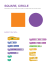More information

### What you can do with a V7 chord: Declaring Dominants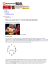More information

### Root Position 3-Note Chords www.JasonWerkema.com (for 6-string Bass)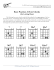More information

### fire stone home products outback chair company laneventure glosterMore information

### Name: __________________________________________________________________ Period: ___________________More information

### 12-2 Practice Chords and Arcs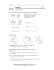More information

### O Holy NightMore information

### Downloaded from by guest on September 11, 2014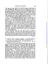More information

### C G G G F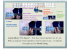More information

### NOMENCLATURE LEGEND: + or ß = raise 1/2 step; — or ∫...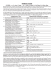More information